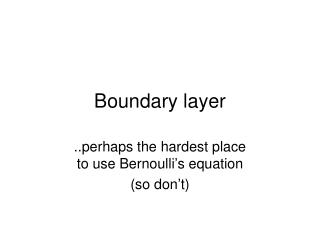Download PresentationBoundary layer

# Boundary layer - PowerPoint PPT Presentation

Boundary layer. ..perhaps the hardest place to use Bernoulli’s equation (so don’t). Drag on a surface – 2 types. Pressure stress / form drag Shear stress / skin friction drag A boundary layer forms due to skin friction. Boundary layer – velocity profile.I am the owner, or an agent authorized to act on behalf of the owner, of the copyrighted work described.
Download Presentation## Boundary layer

Download Policy: Content on the Website is provided to you AS IS for your information and personal use and may not be sold / licensed / shared on other websites without getting consent from its author.While downloading, if for some reason you are not able to download a presentation, the publisher may have deleted the file from their server.

- - - - - - - - - - - - - - - - - - - - - - - - - - E N D - - - - - - - - - - - - - - - - - - - - - - - - - -
Presentation Transcript
1. Boundary layer ..perhaps the hardest place to use Bernoulli’s equation (so don’t)

2. Drag on a surface – 2 types • Pressure stress / form drag • Shear stress / skin friction drag • A boundary layer forms due to skin friction

3. Boundary layer – velocity profile • Far from the surface, the fluid velocity is unaffected. • In a thin region near the surface, the velocity is reduced • Which is the “most correct” velocity profile? …this is a good approximation near the “front” of the plate

4. Boundary layer growth • The free stream velocity is u0, but next to the plate, the flow is reduced by drag • Farther along the plate, the affect of the drag is felt by more of the stream, and because of this • The boundary layer grows

5. Boundary layer transition • At a certain point, viscous forces become to small relative to inertial forces to damp fluctuations • The flow transitions to turbulence • Important parameters: • Viscosity μ, density ρ • Distance, x • Velocity UO • Reynolds number combines these into one number

6. First focus on “laminar” boundary layer • A practical “outer edge” of the boundary layer is where u = uo x 99% • Across the boundary layer there is a velocity gradient du/dy that we will use to determine τ

7. Let’s look at the growth of the boundary layer quantitatively.

8. The velocity profiles grow along the surface • What determines the growth rate and flow profile?

9. Blasius δ(x) • Resistance of fluid to change in velocity due to viscous forces depends on…? • Velocity, UO • Viscosity, μ • Density, ρ • Position, x • The Reynolds number • Blasius (1908) derived:

10. B L thickness & fluid properties

11. Blasius, cont’d: Surface shear stress a • Shear stress δ(x)

12. Boundary layer transition • At a certain point, viscous forces become to small relative to inertial forces to damp fluctuations • The flow transitions to turbulence • Important parameters: • Viscosity μ, density ρ • Distance, x • Velocity UO • Reynolds number combines these into one number

13. How does BL transition?

14. Next focus on “turbulent” boundary layer • A practical “outer edge” of the boundary layer is (still) where u = uo x 99% • Across the boundary layer there is a velocity gradient as well as variations in the velocity that determine τ

15. Turbulence: average & fluctuating velocity The velocity profile concerns the mean velocity. The fluctuating part contributes to the internal stress for high Re flow.

16. Three zones in the turbulent BL

17. Viscous sub-layer • The velocity in the viscous sub-layer is linear

18. Log law of the wall

19. Majority of the boundary layer • 105 < Re < 107

20. Laminar, Turbulence, Induced Turbulence

21. Example 9.8 from text Assume that a boundary layer over a smooth, flat plate is laminar at first and then becomes turbulent at a critical Reynolds number of 5 x 105. If we have a plate 3 m long x 1 m wide, and if air at 20°C and atmospheric pressure flows past this plate with a velocity of 30 m/s what will be the average resistance coefficient Cf for the plate? Also, what will be the total shearing resistance of one side of the plate and what will be the resistance due to the turbulent part and the laminar part of the boundary layer? What is the answer to the same questions if the boundary layer is “tripped” by some sufficiently large roughness element that the boundary layer is turbulent from the beginning?

22. Example